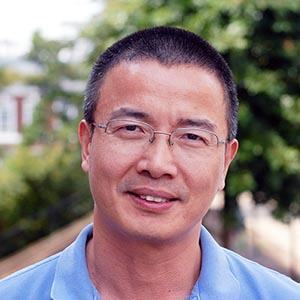ContentResearch interests:

Representation Theory; Connections to Algebraic Combinatorics and Algebraic Geometry

My papers in arXiv

I have research interests in the following subjects:

• Quantum groups, i-quantum groups, and canonical bases
• Hecke algebras: finite, affine, and spin variants
• (Spin) symmetric groups and algebraic q-combinatorics
• Infinite-dimensional Lie algebras and vertex algebras
• (Super) category O and Kazhdan-Lusztig theory
• Representation theory of Lie superalgebras
• Geometric representation theory
• Modular representation theory
• Categorification
• (More keywords: Schur duality, Howe duality, McKay correspondence, Hilbert schemes of points)

I am on the Editorial Board of the following journals: